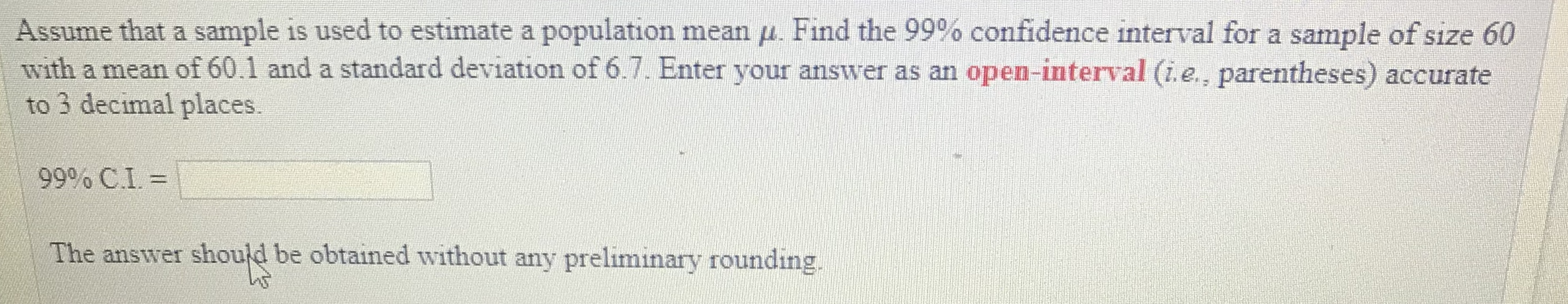# Assume that a sample is used to estimate a population mean μ Find the 99% confidence interval for a sample ofsıze 60with a mean of 60.1 and a standard deviation of 6.7. Enter your answer as an open-interval (i.e., parentheses) accurateto 3 decimal places.99% CI =he answer should be obtained without any preliminary rounding,

Question
147 viewshelp_outlineImage TranscriptioncloseAssume that a sample is used to estimate a population mean μ Find the 99% confidence interval for a sample ofsıze 60 with a mean of 60.1 and a standard deviation of 6.7. Enter your answer as an open-interval (i.e., parentheses) accurate to 3 decimal places. 99% CI = he answer should be obtained without any preliminary rounding, fullscreen
check_circle

Step 1

Find the distribution that has to be used to construct the confidence interval in the given situation:

It is given that, a sample of size 60 with mean 60.1 and standard deviation 6.7 is used to estimate the population mean.

The sample size is n = 60,

The sample mean is x–bar = 60.1,

The sample standard deviation is s  = 6.7.

The required conditions for using t distribution to estimate the confidence interval about mean is given below:

• The population standard deviation σ must be unknown.
• Either the sample size (n) must be greater than 30 or the population must be normally distributed.

Here, the population standard deviation is unknown. The data just tells about the sample standard deviation.

Moreover the sample size is n = 60 (Which is greater than 30).

Hence, the two conditions are satisfied.

Thus, the t–distribution is used to construct the confidence interval for the population mean in the given situation.

The approximate 100*(1–α)% confidence interval for the population mean will be obtained using the formula given below:

Step 2

Obtain the critical value:

The required confidence level is 100*(1–α)% = 99%.

From the given common t-values using t-distribution the critical value is obtained as 2.662 from the calculation given below:

Step 3

Find the 95% confidence interval for the population mean:

Here, n = 60, x–bar = 60.1, s = 6.7.

The critical value is t(α/2, n–1) = t0.005,59 = 2.662.

Denote the population mean as µ.

The 95%...

### Want to see the full answer?

See Solution

#### Want to see this answer and more?

Solutions are written by subject experts who are available 24/7. Questions are typically answered within 1 hour.*

See Solution
*Response times may vary by subject and question.
Tagged in

### Other